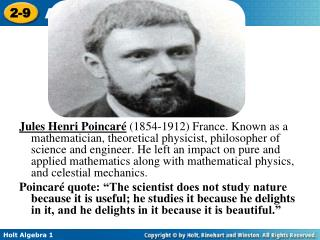Download PresentationWarm Up 1. Write 0.03 as a percent. 2. Write as a decimal.

# Warm Up 1. Write 0.03 as a percent. 2. Write as a decimal. - PowerPoint PPT Presentation

Jules Henri Poincaré (1854-1912) France. Known as a mathematician, theoretical physicist, philosopher of science and engineer. He left an impact on pure and applied mathematics along with mathematical physics, and celestial mechanics.I am the owner, or an agent authorized to act on behalf of the owner, of the copyrighted work described.
Download Presentation## Warm Up 1. Write 0.03 as a percent. 2. Write as a decimal.

Download Policy: Content on the Website is provided to you AS IS for your information and personal use and may not be sold / licensed / shared on other websites without getting consent from its author.While downloading, if for some reason you are not able to download a presentation, the publisher may have deleted the file from their server.

- - - - - - - - - - - - - - - - - - - - - - - - - - E N D - - - - - - - - - - - - - - - - - - - - - - - - - -
Presentation Transcript
1. Jules Henri Poincaré(1854-1912) France. Known as a mathematician, theoretical physicist, philosopher of science and engineer. He left an impact on pure and applied mathematics along with mathematical physics, and celestial mechanics. Poincaré quote: “The scientist does not study nature because it is useful; he studies it because he delights in it, and he delights in it because it is beautiful.”

2. Warm Up 1. Write 0.03 as a percent. 2. Write as a decimal. Find each value. Round to the nearest tenth if necessary. 3. Find 10% of 53. 3% 0.045 5.3

3. Objectives Use common applications of percents. Estimate with percents.

4. Caution! You must convert a percent to a decimal or a fraction before doing any calculations with it.

5. Example 1: Business Application Mr. Cortez earns a base salary of \$26,000 plus a sales commission of 5%. His total sales for one year were \$300,000. Find his total pay for the year. total pay = base salary + commission Write the formula for total pay. = base + % of total sales Write the formula for commission. = 26,000 + 5% of 300,000 Substitute values given in the problem. Write the percent as a decimal. = 26,000 + (0.05)(300,000) = 26,000 + 15,000 Multiply. = 41,000 Add. Mr. Cortez’s total pay was \$41,000.

6. Check It Out! Example 1 A telemarketer earns \$350 per week plus 12% commission on sales. Find her total pay for a week in which her sales were \$940. total pay = base salary + commission Write the formula for total pay. = base + % of total sales Write the formula for commission. = 350 + 12% of 940 Substitute values given in the problem. Write the percent as a decimal. = 350 + (0.12)(940) = 350 + 112.80 Multiply. = 462.80 Add. The telemarketer’s total pay was \$462.80.

7. P t I = r Time in years Simple interest Interest rate per year as a decimal Principal Interest is the amount of money charged for borrowing money, or the amount of money earned when saving or investing money. Principal is the amount borrowed or invested. Simple interest is interest paid only on the principal. Simple Interest Paid Annually

8. Example 2A: Financial Application Find the simple interest paid for 3 years on a \$2500 loan at 11.5% per year. I = Prt Write the formula for simple interest. Substitute known values. Write the interest rate as a decimal. I = (2500)(0.115)(3) I = 862.50 Multiply. The amount of interest is \$862.50.

9. Multiply 5000 .. Since r is multiplied by 2500, divide both sides by 2500 to undo the multiplication. Example 2B: Financial Application After 6 months, the simple interest earned on an investment of \$5000 was \$45. Find the interest rate. Write the formula for simple interest. I = Prt Substitute the given values. 45 = 2500r 0.018 = r The interest rate is 1.8%.

10. Helpful Hint When you are using the formula I= Prt to find simple interest paid annually, t represents time in years. One month is year.

11. Check It Out! Example 2a Find the simple interest earned after 2 years on an investment of \$3000 at 4.5% interest earned annually. Write the formula for simple interest. I = Prt I = (3000)(0.045)(2) Substitute the given values. Multiply. I = 270 The interest earned is \$270.

12. 306 = (P)(0.08) 306 =.04P Multiply 0.08 . Since P is multiplied by 0.04 , divide both sides by 0.04. Check It Out! Example 2b The simple interest paid on a loan after 6 months was \$306. The annual interest rate was 8%. Find the principal. Write the formula for simple interest. I = Prt Substitute the given values. 7650 = P The remaining principal is \$7650.

13. Practice 1. A computer salesperson earns a base salary of \$32,000 per year plus a commission of 6% on total sales. Total sales for one year were \$350,000. Find the salesperson’s total pay for the year. \$53,000 2. Find the simple interest paid for 9 months on a a \$500 loan at 8% per year. \$30 3. After 2 years the simple interest earned on an investment of 4000 was \$216. Find the interest rate. 2.7%

14. Practice 4. A family’s dinner check was \$38.82. Estimate a 15% tip. About \$6.00 5.The sales tax rate is 7.1%. Estimate the sales tax on a television set that costs \$399. About \$28